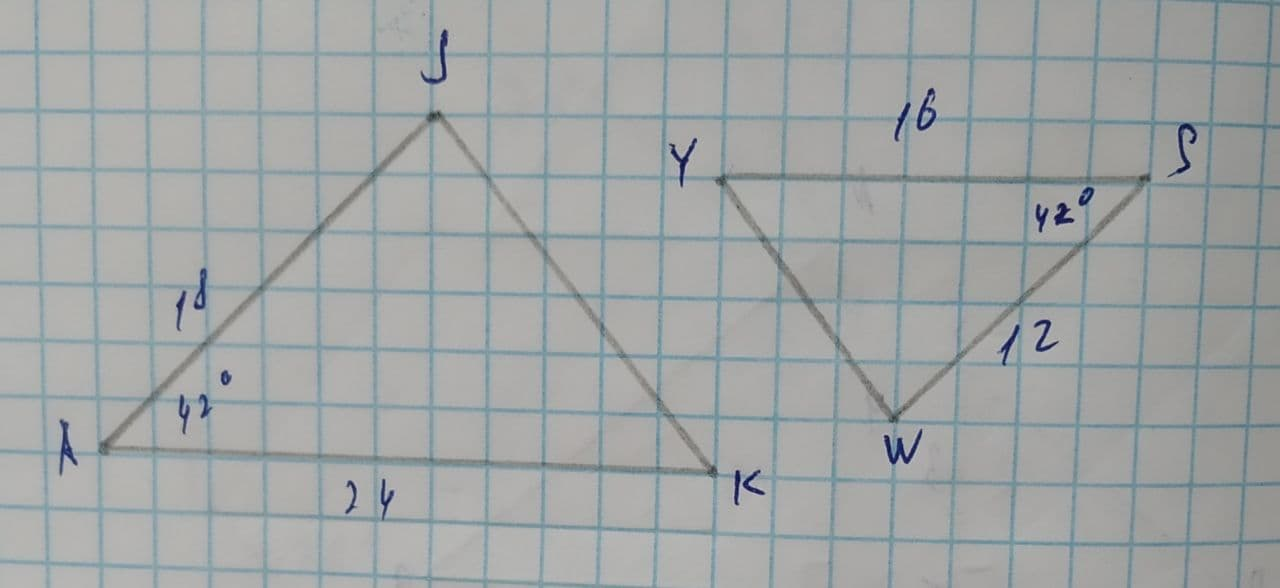Question# \triangle JAK\sim \triangle Determine if the triangles are similar.

Similarity
ANSWEREDDetermine if the triangles are similar.
If they are, complete the similarity statement and indicate the theorem that proves similarity.
If they are not similar, select "not similar" for both entries.$$\displaystyle\triangle{J}{A}{K}\sim\triangle$$? by ?2021-08-01
Step 1
Given,
The triangle JKA with JA = 18. AK=24 and $$\displaystyle\angle{A}={42}^{{\circ}}$$
The triangle WSY with WS = 12. SY=16 and $$\displaystyle\angle{S}={42}^{{\circ}}$$
We have to find the similarity between the triangles.
Step 2
In $$\displaystyle\triangle{J}{A}{K}\ {\quad\text{and}\quad}\ \triangle{W}{S}{Y}$$
$$\displaystyle{\frac{{{J}{A}}}{{{W}{S}}}}={\frac{{{18}}}{{{12}}}}={\frac{{{3}}}{{{2}}}}$$
$$\displaystyle{\frac{{{A}{k}}}{{{S}{Y}}}}={\frac{{{24}}}{{{16}}}}={\frac{{{3}}}{{{2}}}}$$
$$\displaystyle\angle{A}=\angle{S}={42}^{{\circ}}$$
So,
By SAS(side-angle-side) rule of similarity
$$\displaystyle\triangle{J}{A}{K}\sim\triangle{W}{S}{Y}$$